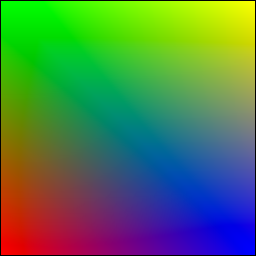# The hard way¶

Before even using OpenGL, we need to open a window with a valid GL context. This can be done using toolkit such as Gtk, Qt or Wx or any native toolkit (Windows, Linux, OSX). Note there also exists dedicated toolkits such as GLFW or GLUT and the advantage of GLUT is that it’s already installed alongside OpenGL. Even if it is now deprecated, we’ll use GLUT since it’s a very lightweight toolkit and does not require any extra package. Here is a minimal setup that should open a window with garbage on it (since we do not even clear the window):

```import sys
import ctypes
import numpy as np
import OpenGL.GL as gl
import OpenGL.GLUT as glut

def display():
glut.glutSwapBuffers()

def reshape(width,height):
gl.glViewport(0, 0, width, height)

def keyboard( key, x, y ):
if key == '\033':
sys.exit( )

glut.glutInit()
glut.glutInitDisplayMode(glut.GLUT_DOUBLE | glut.GLUT_RGBA)
glut.glutCreateWindow('Hello world!')
glut.glutReshapeWindow(512,512)
glut.glutReshapeFunc(reshape)
glut.glutDisplayFunc(display)
glut.glutKeyboardFunc(keyboard)
glut.glutMainLoop()
```

The glutInitDisplayMode tells OpenGL what are the context properties. At this stage, we only need a swap buffer (we draw on one buffer while the other is displayed) and we use a full RGBA 32 bits color buffer (8 bits per channel).

Note

GLUT is now deprecated and you might prefer to use GLFW which is actively maintained.

## Building the program¶

First we need to build a program that link a vertex and a fragment shader. Building such program is relatively straightforward provided we do not check for errors. First we need to request program and shader slots from GPU:

```program  = gl.glCreateProgram()
```

Then we can compile shaders’ code into GPU objects:

```vertex_code = """
uniform float scale;
attribute vec2 position;
attribute vec4 color;
varying vec4 v_color;
void main()
{
gl_Position = vec4(scale*position, 0.0, 1.0);
v_color = color;
} """

fragment_code = """
varying vec4 v_color;

void main()
{
gl_FragColor = v_color;
} """

# Set shaders source

```

We can now build and link the program:

```gl.glAttachShader(program, vertex)
```

We can not get rid of shaders, they won’t be used again:

```gl.glDetachShader(program, vertex)
```

Finally, we make program the default program to be ran. We can do it now because we’ll use a single in this example:

```gl.glUseProgram(program)
```

## Building the buffer¶

Next, we need to build a buffer that will be used to transfer vertices from CPU to GPU memory. Building a buffer is quite simple:

```# Request a buffer slot from GPU
buffer = gl.glGenBuffers(1)

# Make this buffer the default one
gl.glBindBuffer(gl.GL_ARRAY_BUFFER, buffer)

gl.glBufferData(gl.GL_ARRAY_BUFFER, data.nbytes, data, gl.GL_DYNAMIC_DRAW)
```

## Binding the buffer to the program¶

Next, we need to bind the buffer to the program and this requires some computations. We need to tell the GPU how to read the buffer and bind each value to the relevant attribute. To do this, GPU needs to kow what is the stride between 2 consecutive element and what is the offset to read one attribute:

```stride = data.strides

offset = ctypes.c_void_p(0)
loc = gl.glGetAttribLocation(program, "position")
gl.glEnableVertexAttribArray(loc)
gl.glBindBuffer(gl.GL_ARRAY_BUFFER, buffer)
gl.glVertexAttribPointer(loc, 3, gl.GL_FLOAT, False, stride, offset)

offset = ctypes.c_void_p(data.dtype["position"].itemsize)
loc = gl.glGetAttribLocation(program, "color")
gl.glEnableVertexAttribArray(loc)
gl.glBindBuffer(gl.GL_ARRAY_BUFFER, buffer)
gl.glVertexAttribPointer(loc, 4, gl.GL_FLOAT, False, stride, offset)
```

Here we’re basically telling the program how to bind data to the relevant attribute. This is made by providing the stride of the array (how many bytes between each record) and the offset of a given attribute.

## Binding the uniform¶

Finally, we also need to bind the uniform which is much more simpler. We request the location of the uniform and we upload the value using the dedicated function to upload one float only:

```loc = gl.glGetUniformLocation(program, "scale")
gl.glUniform1f(loc, 1.0)
```

We’re almost ready to render something but let’s first fill some values:

```data['color']    = [ (1,0,0,1), (0,1,0,1), (0,0,1,1), (1,1,0,1) ]
data['position'] = [ (-1,-1),   (-1,+1),   (+1,-1),   (+1,+1)   ]
```

If the color field makes sense (normalized RGBA values), why do we use coordinates such as (-1,-1) for vertex position ? We know the windows size is 512x512 pixels in our case, so why not use (0,0) or (512,512) instead ?

At this point in the tutorial, OpenGL does not really care of the actual size of the window (also called viewport) in terms of pixels. If you look at the GLUT code above, you may have noticed this line:

```def reshape(width,height):
gl.glViewport(0, 0, width, height)
```

This function is called whenever the window is resized and the glViewport call does two things. It instructs OpenGL of the current window size and it setup an implicit normalized coordinate system that goes from (-1,-1) (for the bottom-left corner) to (+1,+1) to top-right corner. Thus, our vertices position cover the whole window.

## Rendering¶

Before rendering, we need to tell OpenGL what to do with our vertices, i.e. what does these vertices describe in term of geometrical primitives. This is quite an important parameter since this determines how many fragments will be actually generated by the shape as illustrated on the image below:There exist other primitives but we won’t used them during this tutorial (and they’re mainly related to geometry shaders that are not introduced in this tutorial). Since we want do display a square, we can use 2 triangles to make a square and thus we’ll use a `GL_TRIANGLE_STRIP` primitive. We’ll see later how to make more complex shapes.Ok, we’re done, we can now rewrite the display function:

```def display():
gl.glClear(gl.GL_COLOR_BUFFER_BIT)
gl.glDrawArrays(gl.GL_TRIANGLE_STRIP, 0, 4)
glut.glutSwapBuffers()
```

The 0, 4 arguments in the glDrawArrays tells OpenGL we want to display 4 vertices from our array and we start at vertex 0.

Full source code is available on github